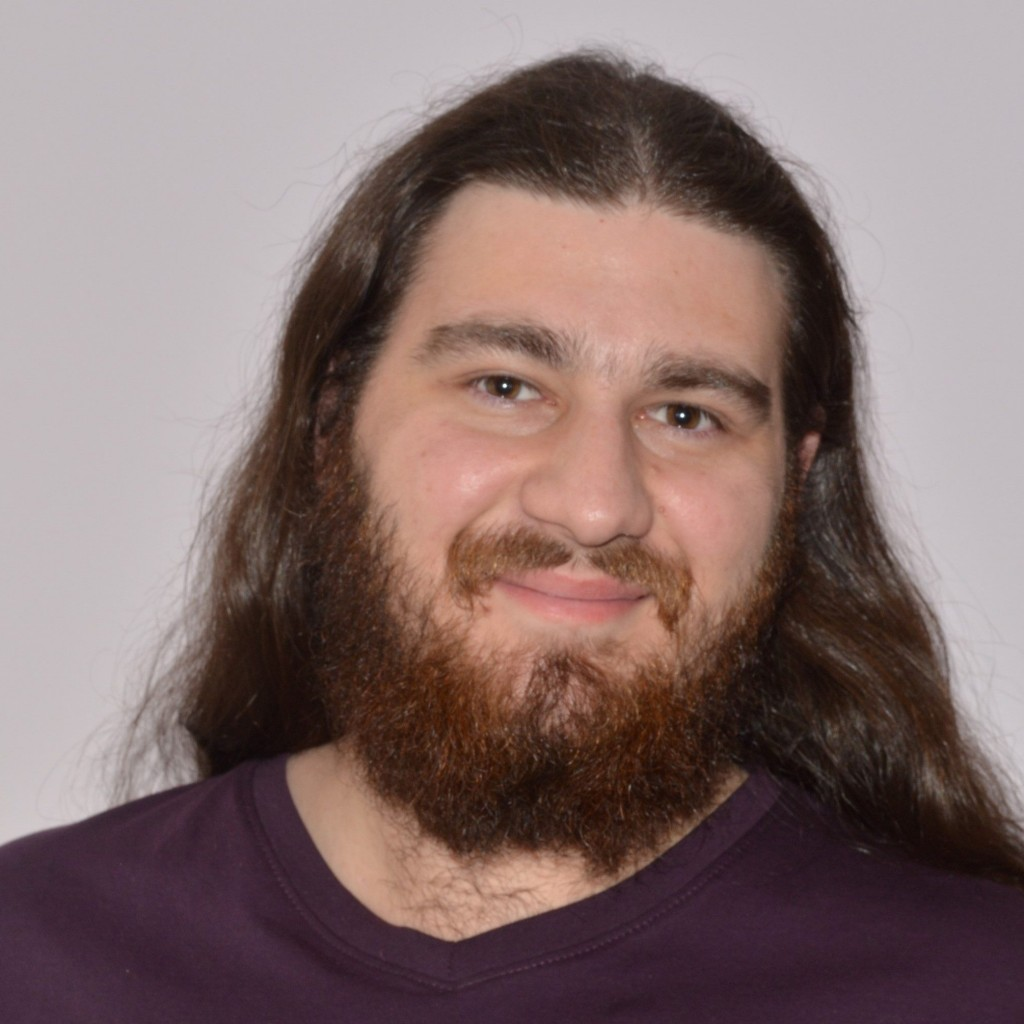Throughout this project I studied Fredholm operators (a certain subset of bounded linear operators) and used them to study the solutions of differential equations. Not only did I learn about and develop intuition for a field with potential for further research but I also got an idea for what in particular I am looking for in a career in mathematics.

In mathematical Industry and Academia virtually all jobs that pure mathematicians do can be split into two ‘sides’; developing new tools with niche areas of maths, and using these tools to solve problems. While this is a gross oversimplification, and is certainly not a clean divide, I believe this is a good starting point for young maths students to decide what they want to do after university and for me in particular, led me to choose this project.

The main focus of my project was to develop my understanding of Fredholm operators and using them, alongside some degree theory, to show that certain equations have a solution. This is essentially both ‘sides’ of working in the pure maths field, learning and building tools from a niche area of maths (Fredholm operators and Degree Theory) and using said tools to try and solve equations (or in this case, prove existence of solutions to equations).

Throughout my time working on this project I did indeed develop some necessary tools involving Fredholm operators and gained some insight into the history of degree theory that led up to developing a degree for Fredholm operators (such as a degree for finite-dimensional operators, or infinite-dimensional operators of a certain type, etc.). Then I studied the alternate ways that these degrees can be defined, which do vary considerably, and how they can be used.

For example, if we look at trying to find existence of a solution for the integral kernel operator  for any function f in L­2 we can show using the Leray-Schauder degree that showing this is equivalent to proving existence of a solution for when f=0, which is a much easier task and in my opinion a really cool result to fall out of all the complicated maths.

To sum up, while this project did what I originally intended it to do and helped me decide which ‘side’ of pure maths I want to work on,  it also let me learn about a topic with a large potential for further research and gave me a taste of what being in Academia is like.

Dion Nikolic
University of WollongongView Dion Nikolic
Profile